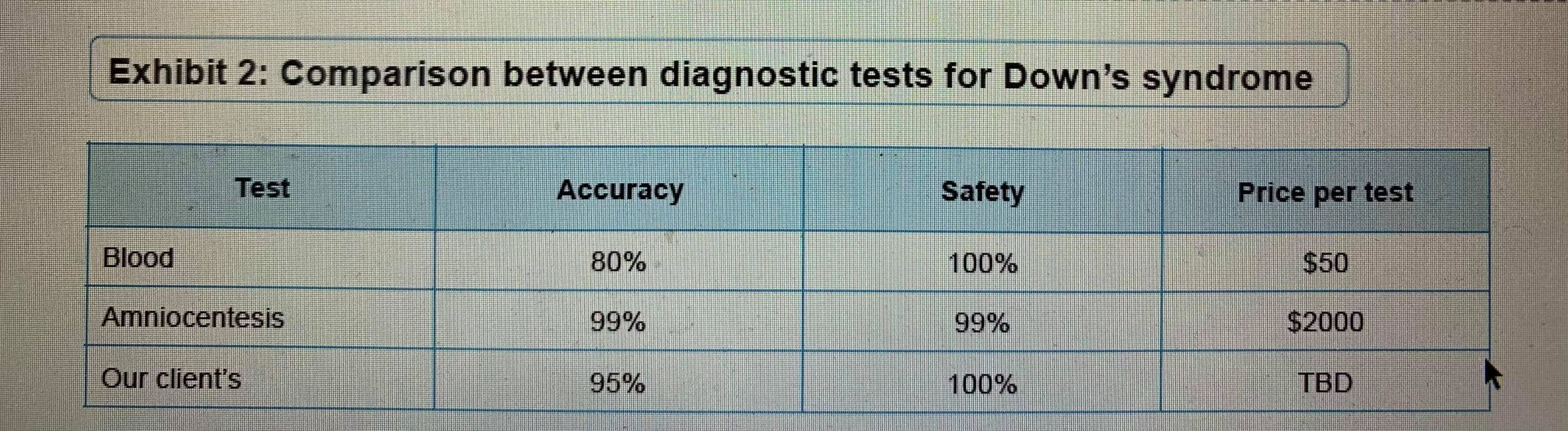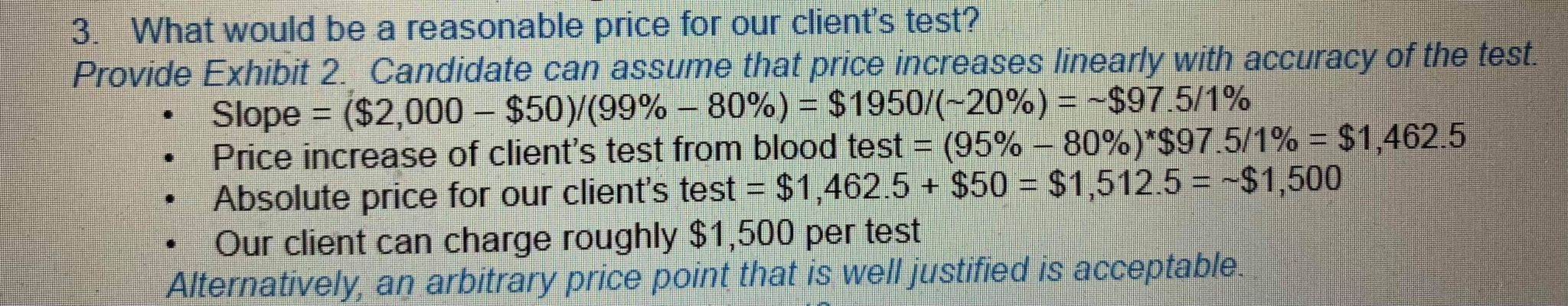# Calculating price

case math
New answer on Aug 02, 2021
109 Views

I'm not sure how they are calculating price here. I got the slope part, but after that? It's not a y=slope*x + b formula? Can we simplify this and just assume that since the accuracy is closer to 99%, our price should be closer to 2k, and do a proportional decrease (i.e. 2000 * 95%/99%= \$1920?)• Date ascending
• Date descending

Hey there,

Think about the solution like this:

• Each percaentage point of additional accuracy after 80% (blod test accuracy) worth \$97.5 (your slope)
• Our clients, test has 15 pp more than the blod test (95%-88%), so it has an additional value of: \$97.5*15 = \$1462.5
• The first 80% accuracy points worth 50\$ as in the blood test - the additional 15% points worth \$1,462.5 as calculated above
• Total price = \$1,462.5 + \$50